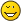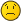complex iteration (complex "height")GottfriedUltimate FellowPosts: 901 Threads: 130 Joined: Aug 2007 11/14/2007, 09:32 AM (This post was last modified: 11/14/2007, 02:42 PM by Gottfried.) Here I add two plots, which show bases s=2 and base s=sqrt(2), when tetrated to complex heights h. The height-parameter h follows the border of the complex unit-disk, so passes the coordinates (1,0),(0,i),(-1,0),(0,-i ) and is exp(2*Pi*I*x) . Also I have added 4 different scalings of h by the zoom-factor g, where g=1 (h is on the complexunit-circle), g=0.75 (h has radius 0.75), g=0.5 and g=0.25. This is a small picture for s=2:and this a small picture for s=sqrt(2):The curves for value g=1.25 (radius of h=1.25) are partly erratic; a better approximation-method should be able to smooth them a bit. Gottfried Attached Files Thumbnail(s)Gottfried Helms, Kasselbo198214Administrator Posts: 1,624 Threads: 103 Joined: Aug 2007 03/21/2008, 05:21 PM (This post was last modified: 03/21/2008, 05:23 PM by bo198214.) @Gottfried: By which method did you compute your values? Just triggered by the question about bI, I computed it by $bt=\exp_b^{\circ t}(1)$ with $\exp_b^{\circ t}$ being the regular iteration at the lower fixed point of $b^x$ (via the formula given here) for various bases $11$ for which $bI$ is real.GottfriedUltimate FellowPosts: 901 Threads: 130 Joined: Aug 2007 03/22/2008, 01:01 AM bo198214 Wrote:@Gottfried: By which method did you compute your values? Hmm, for whatever reason I didn't find the pari.gp-syntax. I used the diagonalization and took complex powers for the diagonal. Maybe I did it using the square-bell-matrices directly or already using the shifting-to-triangular method, don't remember. What I have are the data for the plots in Excel. Since I'm busy tomorrow (tibet-action) I'll not be able to reproduce the syntax, however I could send you the data if this helps. Everything else I can do on sat evening or sunday, let's see. Gottfried Gottfried Helms, KasselIvarsLong Time FellowPosts: 366 Threads: 26 Joined: Oct 2007 03/22/2008, 09:15 AM (This post was last modified: 03/22/2008, 09:32 AM by Ivars.) @Henryk The next questions to provoke further thinking is : b(i*pi/2)=bln(i)? b(i/2)=? b(-i)=? and at what exact value of b is bi purely real by Your method? @Gottfried What is on axis? What is k?The relation between k and x? Why there is k/64 in expression for h? ... Interesting anyway , Your pictures always seem to contain a lot of information. Ivarsbo198214Administrator Posts: 1,624 Threads: 103 Joined: Aug 2007 03/22/2008, 11:59 AM Ivars Wrote:@Henryk The next questions to provoke further thinking is : b(i*pi/2)=bln(i)? b(i/2)=? b(-i)=? As I am not so interested in particular values perhaps you need to stretch your own brain. The formula I used was: $f^{\circ t}(x)=\lim_{n\to\infty} f^{\circ -n}(a(1-q^t) + q^t f^{\circ n}(x))$, $q=f'(a)$. where $a$ is the attracting fixed point and $f$ is the function to be iterated. Specialized to our case using the lower fixed point $a$ we get the base by $b=a^{1/a}$ and $f'(a)=(b^x)'(a)=\ln(b)b^a=\ln(b)a=\ln(b^a)=\ln(a)$, so: $bz=\exp_b^{\circ z}(1)=\lim_{n\to\infty} \log_b^{\circ n}(a(1-\ln(a)^z) + \ln(a)^z \exp_b^{\circ n}(1))$bo198214Administrator Posts: 1,624 Threads: 103 Joined: Aug 2007 03/22/2008, 12:10 PM Gottfried Wrote:[What I have are the data for the plots in Excel. Since I'm busy tomorrow (tibet-action) I'll not be able to reproduce the syntax, however I could send you the data if this helps. Everything else I can do on sat evening or sunday, let's see. I was only interested whether our results match. But one couldnt see from the graph what $\sqrt{2}I$ is, so I would be glad if you could compare this with my result of $\sqrt{2}I=1.210309011+.5058275611*I$GottfriedUltimate FellowPosts: 901 Threads: 130 Joined: Aug 2007 03/22/2008, 03:25 PM (This post was last modified: 03/22/2008, 11:45 PM by Gottfried.) bo198214 Wrote:I was only interested whether our results match. But one couldnt see from the graph what $\sqrt{2}I$ is, so I would be glad if you could compare this with my result of $\sqrt{2}I=1.210309011+.5058275611*I$ Yepp, that's exactly the value that I've got for height h=I (up to 7'th digit) In my excel-file it is real=1.2103090 imag = 0.50582757 [update] with higher precision I recomputed the value and got in the 92..96 partial-sums the following approximations: Code:´   1.210309025559961+0.5058275713618201*I   1.210309025559961+0.5058275713618201*I   1.210309025559961+0.5058275713618201*I   1.210309025559961+0.5058275713618201*I   1.210309025559961+0.5058275713618201*I ---------------------------------------------   1.210309011      + .5058275611          *I  <--- yours So this differs from the 7'th digit; anyway - I just computed this with the function U_t (x) = t^x - 1 T_b(x) = b^x U_t°h(x) and T_b°h(x) as their iterates of general height h and the shift T_b°h(x) = (U_t°h(x/t-1) +1)*t and the height-iteration by applying powers to the diagonal of the diagonalized operator for U_t(x) with 96 terms, using b=sqrt(2),t=2,x=1 If your values have full accuracy, then there must be a methodical difference, which I would like to find; I'll check today with other fixpoint-shifts. Gottfried Gottfried Helms, KasselGottfriedUltimate FellowPosts: 901 Threads: 130 Joined: Aug 2007 03/22/2008, 03:32 PM (This post was last modified: 03/22/2008, 03:47 PM by Gottfried.) Ivars Wrote:@Gottfried What is on axis? What is k?The relation between k and x? Why there is k/64 in expression for h? ... Interesting anyway , Your pictures always seem to contain a lot of information. Ivarsx-Axis is real, y-axis imaginary value of the result. The complex circle of radius |1| was divided in 64 steps. indicated by k as the number, so each k represents 1/64 of the circumference of the complex unit-circle. But this is only using g=1. I added the other results for equivalent parameters on the complex circles of smaller radii too. So the blue line shows the values for sqrt(2)^^(exp(2*Pi*I*k/64)), k=0..64 the green line for sqrt(2)^^(0.75*exp(2*Pi*I*k/64)), k=0..64 and so on. Here is an updated plot, where the points for h=I (the 16'th point on the blue line) , h=-1 (32'th point) , h=-I (48'th point) are markedGottfried Helms, KasselIvarsLong Time FellowPosts: 366 Threads: 26 Joined: Oct 2007 03/22/2008, 07:45 PM bo198214 Wrote:As I am not so interested in particular values perhaps you need to stretch your own brain. The formula I used was: $f^{\circ t}(x)=\lim_{n\to\infty} f^{\circ -n}(a(1-q^t) + q^t f^{\circ n}(x))$, $q=f'(a)$. where $a$ is the attracting fixed point and $f$ is the function to be iterated. Specialized to our case using the lower fixed point $a$ we get the base by $b=a^{1/a}$ and $f'(a)=(b^x)'(a)=\ln(b)b^a=\ln(b)a=\ln(b^a)=\ln(a)$, so: $bz=\exp_b^{\circ z}(1)=\lim_{n\to\infty} \log_b^{\circ n}(a(1-\ln(a)^z) + \ln(a)^z \exp_b^{\circ n}(1))$ My brain is already overstretched... I think I need some software that can calculate infinitely many logarithmsIvarsbo198214Administrator Posts: 1,624 Threads: 103 Joined: Aug 2007 03/22/2008, 07:49 PM Ivars Wrote:My brain is already overstretched... I think I need some software that can calculate infinitely many logarithmsYa, software might be quite helpful, however no software will compute infinitely many logarithms. Its just a question of approximation. « Next Oldest | Next Newest »

 Possibly Related Threads… Thread Author Replies Views Last Post Real tetration as a limit of complex tetration Daniel 5 547 06/20/2023, 07:52 PM Last Post: tommy1729 Real and complex tetration Daniel 13 2,373 04/04/2023, 10:25 AM Last Post: JmsNxn Evaluating Arithmetic Functions In The Complex Plane Caleb 6 959 02/20/2023, 12:16 AM Last Post: tommy1729 Range of complex tetration as real Daniel 2 845 10/22/2022, 08:08 PM Last Post: Shanghai46 From complex to real tetration Daniel 3 984 10/21/2022, 07:55 PM Last Post: Daniel What are the types of complex iteration and tetration? Daniel 5 1,493 08/17/2022, 02:40 AM Last Post: JmsNxn Complex to real tetration Daniel 1 684 08/14/2022, 04:18 AM Last Post: JmsNxnConvergent Complex Tetration Bases With the Most and Least Imaginary Parts Catullus 0 597 07/10/2022, 06:22 AM Last Post: Catullus Complex to real tetration via Kneser Daniel 3 1,336 07/02/2022, 02:22 AM Last Post: Daniel Real and complex tetration Daniel 3 1,198 06/22/2022, 11:16 PM Last Post: tommy1729

Users browsing this thread: 1 Guest(s)